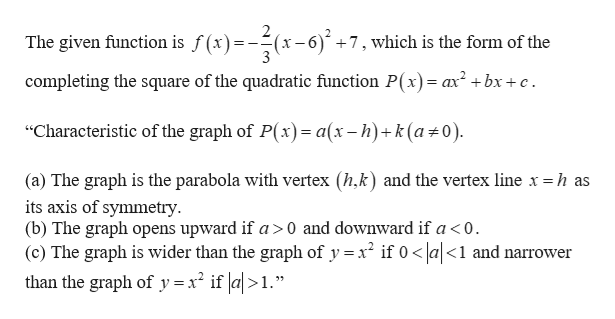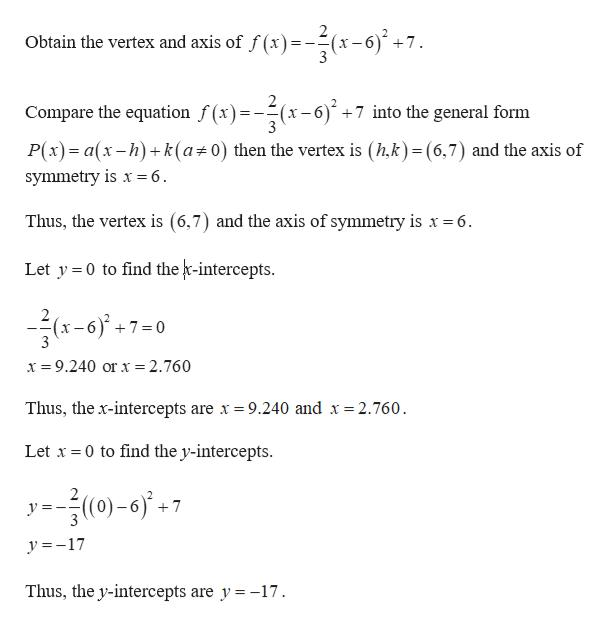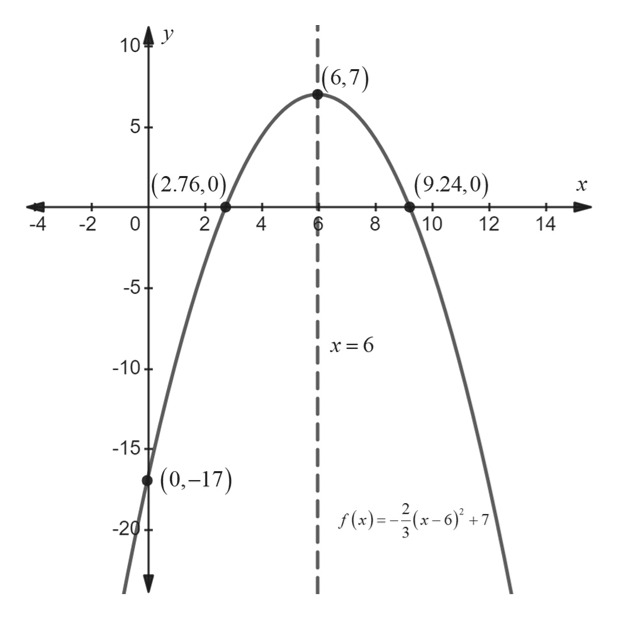# Graph the quadratic function. Give the vertex, axis, x-intercepts, y-intercept, domain, range, and largest open intervals of the domain over which each function is increasing or decreasing.f(x)= -2/3(x-6)2+7

Question
20 views

Graph the quadratic function. Give the vertex, axis, x-intercepts, y-intercept, domain, range, and largest open intervals of the domain over which each function is increasing or decreasing.f(x)= -2/3(x-6)2+7

check_circle

Step 1help_outlineImage TranscriptioncloseThe given function is f(x)=--(x-6) +7, which is the form of the completing the square of the quadratic function P(x) = ax2+bx + c "Characteristic of the graph of P(x)= a(x-h)+k (a#0) (a) The graph is the parabola with vertex (h,k) and the vertex line x = h as its axis of symmetry (b) The graph opens upward if a>0 and downward if a < 0. (c) The graph is wider than the graph of y x if 0a<1 and narrower than the graph of y = x2 if a>1." fullscreen
Step 2help_outlineImage TranscriptioncloseObtain the vertex and axis of f (x)-(x-6) + 7 Compare the equation f(x)(x-6)+7 into the general form P(x) a(x-h)k (a 0) then the vertex is (hk) (6,7) and the axis of symmetry is x= 6 Thus, the vertex is (6,7) and the axis of symmetry is x = 6 Let y 0 to find the r-intercepts. 7 0 x 9.240 or x = 2.760 Thus, the x-intercepts are x = 9.240 and x 2.760. Let x 0 to find the y-intercepts. 2 уз (0)-6)' +7 y 17 Thus, the y-intercepts are y = -17 fullscreen
Step 3

Use the above information to sketch ...help_outlineImage Transcriptionclosei (6,7) 104 (2.76,0 (9.24,0) -4 -2 0 2 4 10 12 14 -5+ x= 6 -10 -15 (0.-17) f()=x-'+7 -20 CO LO fullscreen

### Want to see the full answer?

See Solution

#### Want to see this answer and more?

Solutions are written by subject experts who are available 24/7. Questions are typically answered within 1 hour.*

See Solution
*Response times may vary by subject and question.
Tagged in

### Other